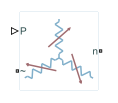Three-phase variable load wired in wye configuration

•Libraries:
Simscape / Electrical / Passive / RLC Assemblies

## Description

The Wye-Connected Variable Load block models a three-phase variable load wired in a wye configuration. Each limb of the load contains a resistor. The block calculates the resistance required to draw the real power of the physical signal input P at the rated voltage that you specify. Therefore, the block can represent a real load.

To ensure that the resistance is always greater than zero, you specify the minimum real power that the load consumes. The minimum real power must be greater than zero.

### Equations

The resistance is defined by

`$R=\frac{{V}_{Rated}^{2}}{P},$`

where:

• R is the per-phase series resistance.

• VRated is the RMS, rated line-line voltage.

• P is the three-phase real power required.

## Ports

### Input

expand all

Physical signal input port associated with the real power.

### Conserving

expand all

Electrical conserving port associated with the neutral phase.

## Parameters

expand all

Whether to model composite or expanded three-phase ports.

Composite three-phase ports represent three individual electrical conserving ports with a single block port. You can use composite three-phase ports to build models that correspond to single-line diagrams of three-phase electrical systems.

Expanded three-phase ports represent the individual phases of a three-phase system using three separate electrical conserving ports.

RMS, rated line-line voltage for the resistance equation.

Minimum real power that the three-phase load dissipates when supplied at the rated voltage. The value must be greater than 0.

## Version History

Introduced in R2014b